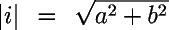# EPA On-line Tools for Site Assessment Calculation

 8 of 67

With data from three points the magnitude and direction of the gradient can be determined. X, Y locations and the measured heads give a matrix for determining the gradient and direction:where a, b, and c are the coefficients of a plane that goes through the three points. The magnitude of the gradient is dermined fromand the angle with North (=0o) is, for example in the first quadrantPrevious Top ^ Next

Home | Glossary | Notation | Links | References | Calculators